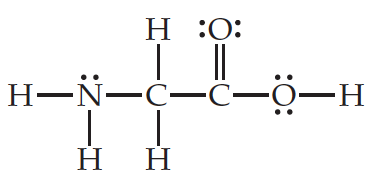# Problem: Consider the Lewis structure for glycine, the simplest amino acid:What is the total number of σ bonds in the entire molecule, and what is the total number of π bonds?

###### FREE Expert Solution

We are asked to identify the total number of σ bonds in the entire molecule, and what is the total number of π bonds

Single bonds → 1 σ bonds

Double bond → 1 σ bonds + 1  π bonds

Triple bond → 1 σ bonds + 2 π bonds

97% (322 ratings)###### Problem Details

Consider the Lewis structure for glycine, the simplest amino acid:What is the total number of σ bonds in the entire molecule, and what is the total number of π bonds?